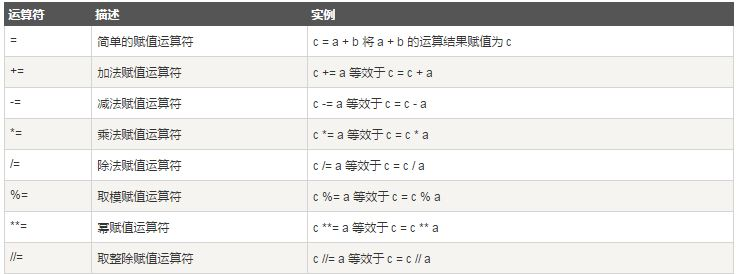# 深入解析python中的变量和赋值运算符

python 变量类型

python中的变量不需要声明，变量的赋值操作既是变量声明和定义的过程。

#!/usr/bin/python
# -*- coding: utf-8 -*-
counter = 100 # 赋值整型变量
miles = 1000.0 # 浮点型
name = “john” # 字符串
print counter
print miles
print name

100
1000.0
john

python允许你同时为多个变量赋值。例如：

a = b = c = 1

a, b, c = 1, 2, “john”

python赋值运算符#!/usr/bin/python
a = 21
b = 10
c = 0
c = a + b
print “line 1 – value of c is “, c
c += a
print “line 2 – value of c is “, c
c *= a
print “line 3 – value of c is “, c
c /= a
print “line 4 – value of c is “, c
c = 2
c %= a
print “line 5 – value of c is “, c
c **= a
print “line 6 – value of c is “, c
c //= a
print “line 7 – value of c is “, c

line 1 – value of c is 31
line 2 – value of c is 52
line 3 – value of c is 1092
line 4 – value of c is 52
line 5 – value of c is 2
line 6 – value of c is 2097152
line 7 – value of c is 99864

Posted in 未分类